Скачать презентацию Fi 8000 Basics of Options Calls Puts Milind

49eede82d34aae669d0f1bfb56a4655d.ppt

• Количество слайдов: 57Fi 8000 Basics of Options: Calls, Puts Milind Shrikhande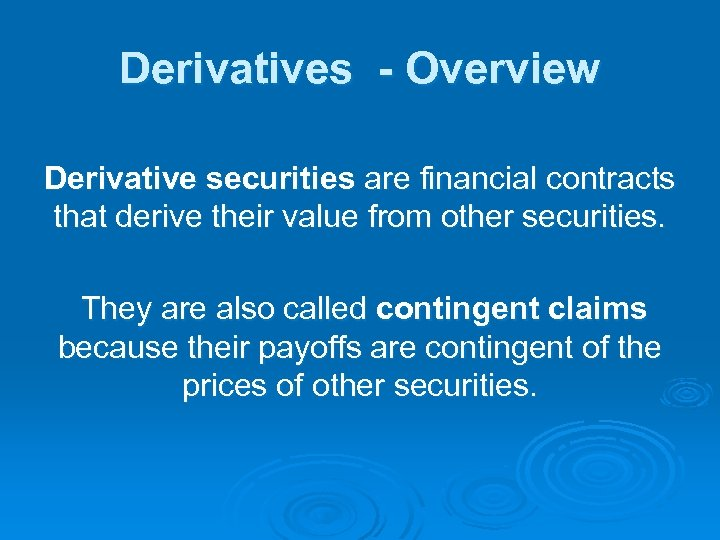Derivatives - Overview Derivative securities are financial contracts that derive their value from other securities. They are also called contingent claims because their payoffs are contingent of the prices of other securities.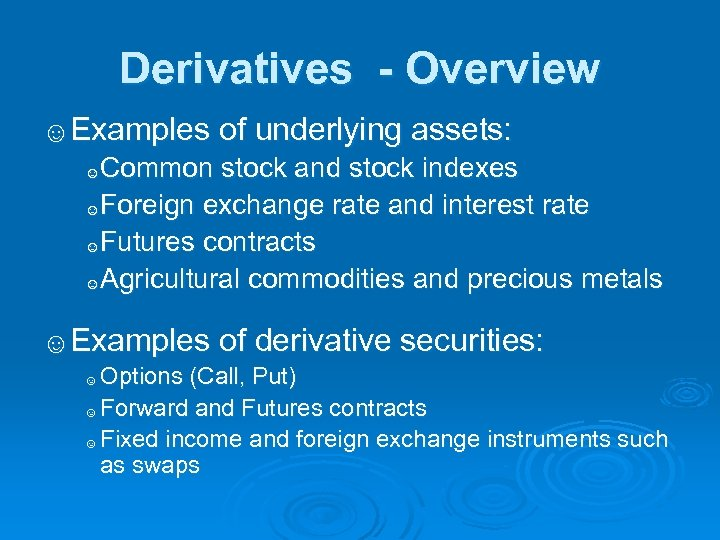Derivatives - Overview ☺Examples of underlying assets: Common stock and stock indexes ☺Foreign exchange rate and interest rate ☺Futures contracts ☺Agricultural commodities and precious metals ☺ ☺Examples of derivative securities: Options (Call, Put) ☺ Forward and Futures contracts ☺ Fixed income and foreign exchange instruments such as swaps ☺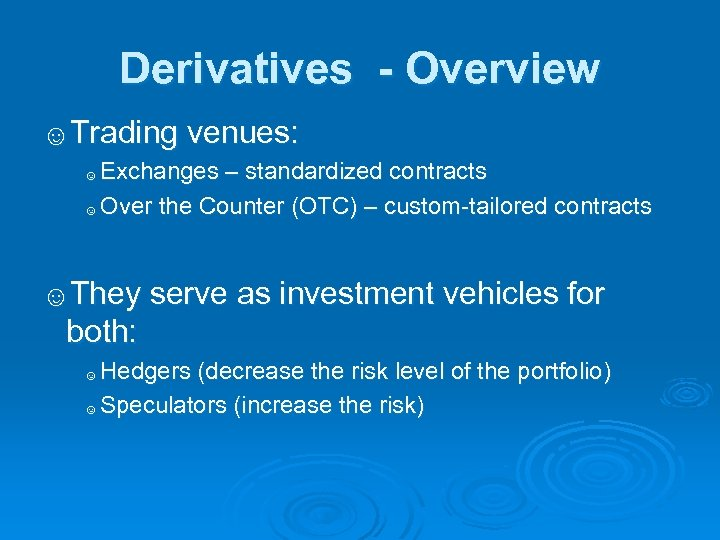Derivatives - Overview ☺Trading venues: Exchanges – standardized contracts ☺ Over the Counter (OTC) – custom-tailored contracts ☺ ☺They serve as investment vehicles for both: Hedgers (decrease the risk level of the portfolio) ☺ Speculators (increase the risk) ☺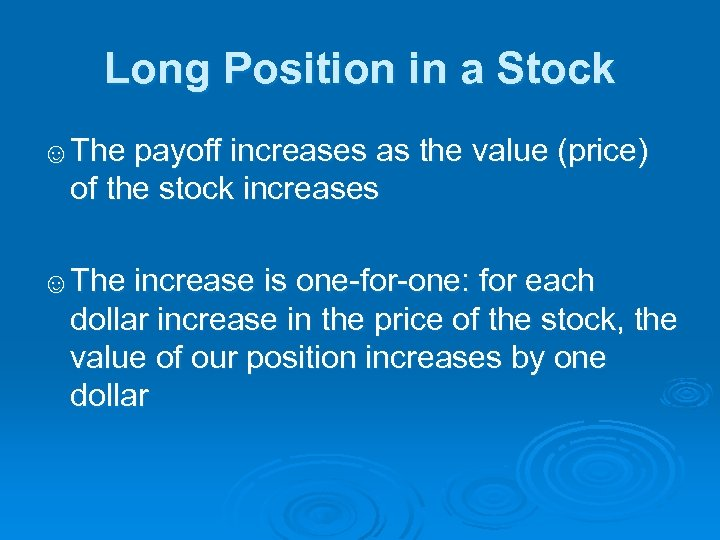Long Position in a Stock ☺The payoff increases as the value (price) of the stock increases ☺The increase is one-for-one: for each dollar increase in the price of the stock, the value of our position increases by one dollar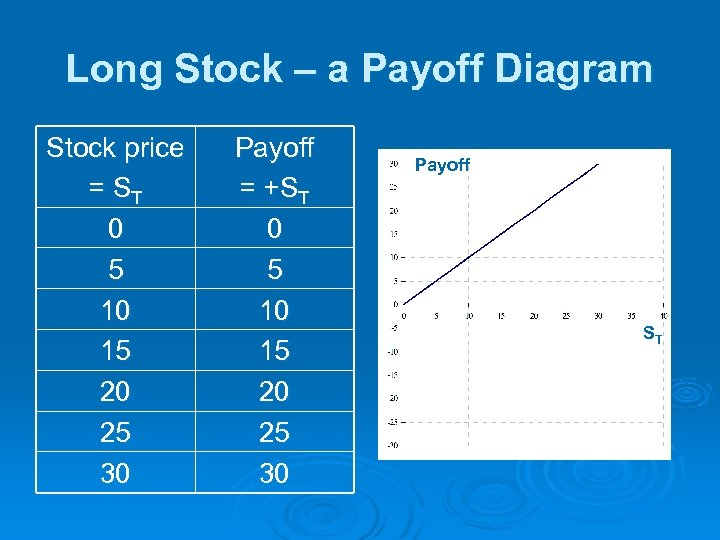Long Stock – a Payoff Diagram Stock price = ST 0 5 10 15 20 25 30 Payoff = +ST 0 5 10 15 20 25 30 Payoff ST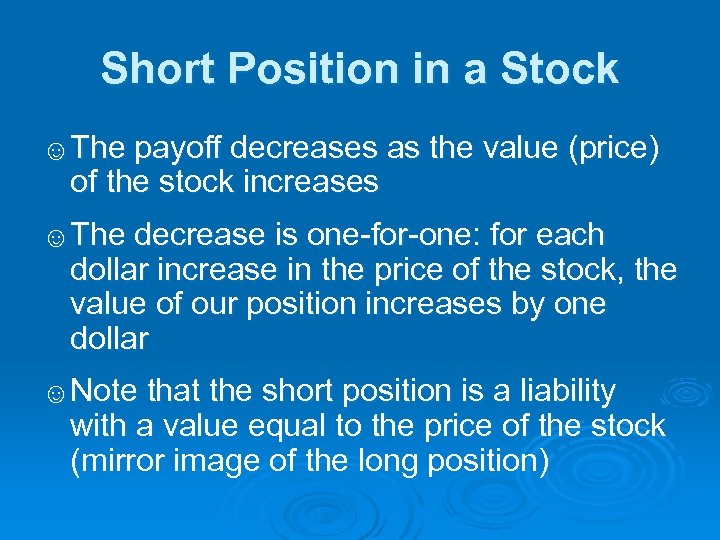Short Position in a Stock ☺The payoff decreases as the value (price) of the stock increases ☺The decrease is one-for-one: for each dollar increase in the price of the stock, the value of our position increases by one dollar ☺Note that the short position is a liability with a value equal to the price of the stock (mirror image of the long position)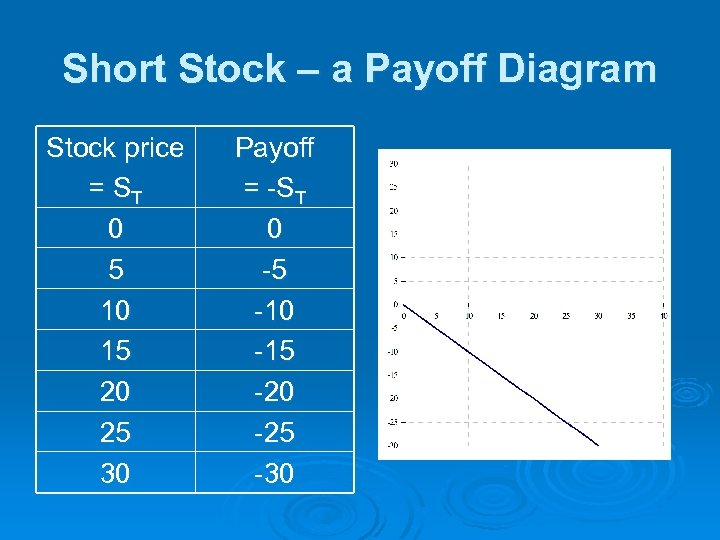Short Stock – a Payoff Diagram Stock price = ST 0 5 10 15 20 25 30 Payoff = -ST 0 -5 -10 -15 -20 -25 -30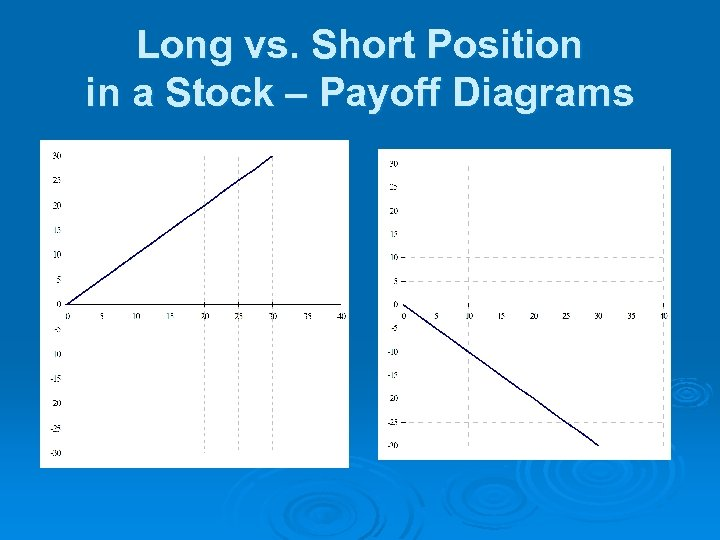Long vs. Short Position in a Stock – Payoff Diagrams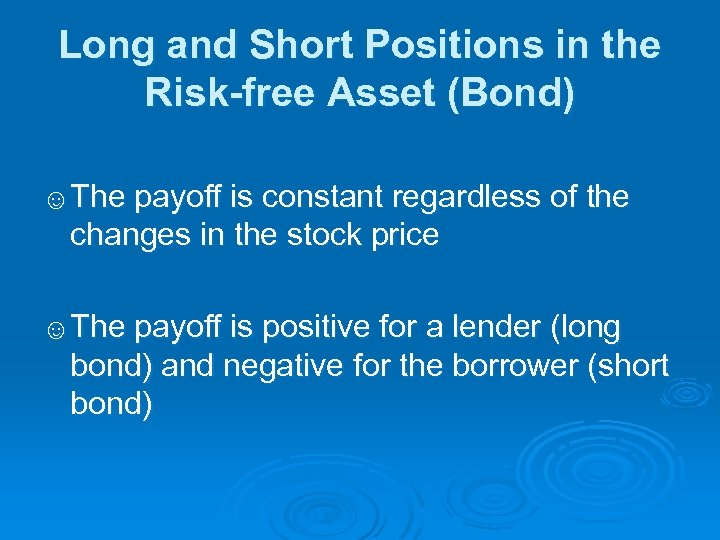Long and Short Positions in the Risk-free Asset (Bond) ☺The payoff is constant regardless of the changes in the stock price ☺The payoff is positive for a lender (long bond) and negative for the borrower (short bond)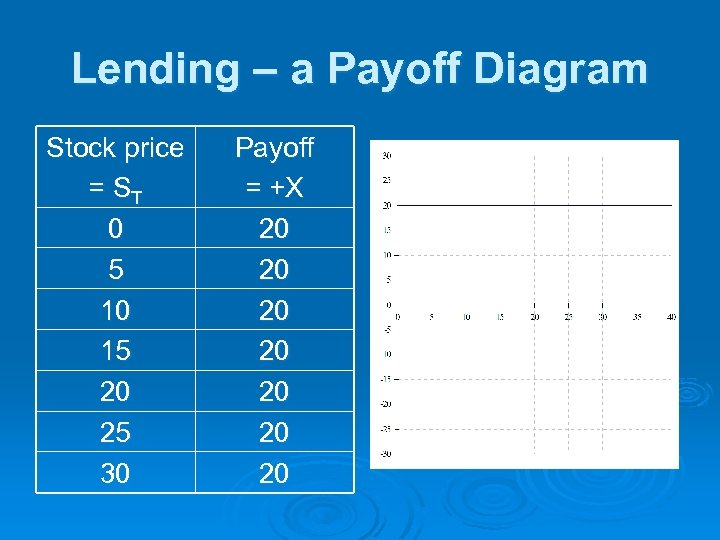Lending – a Payoff Diagram Stock price = ST 0 5 10 15 20 25 30 Payoff = +X 20 20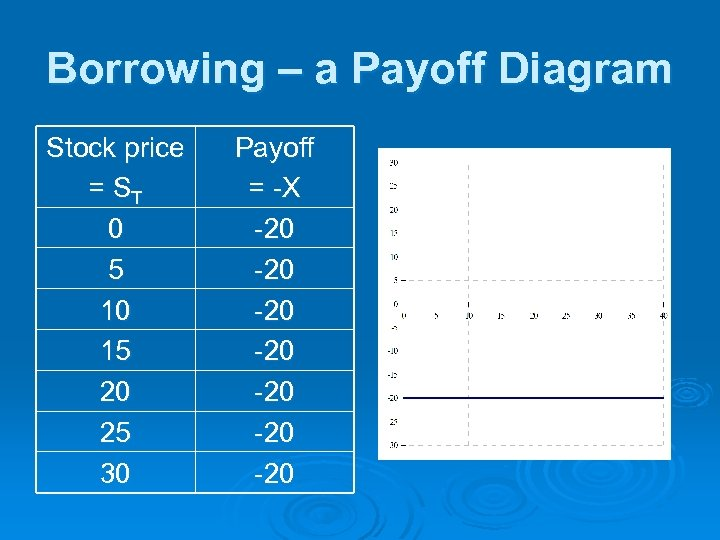Borrowing – a Payoff Diagram Stock price = ST 0 5 10 15 20 25 30 Payoff = -X -20 -20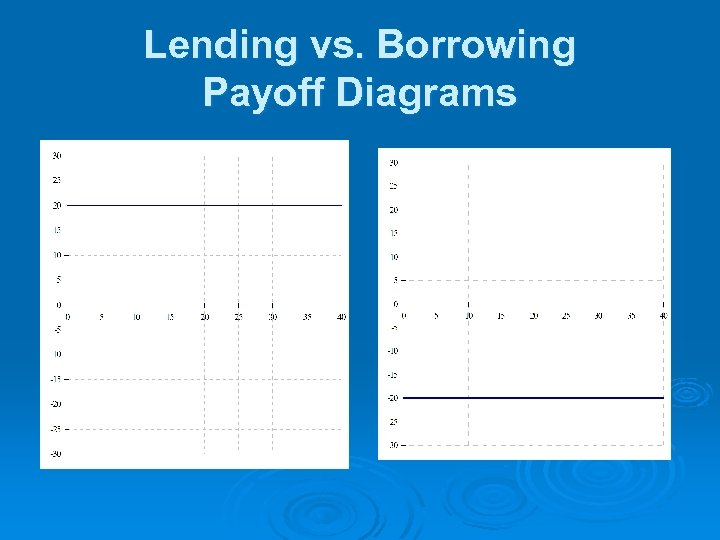Lending vs. Borrowing Payoff Diagrams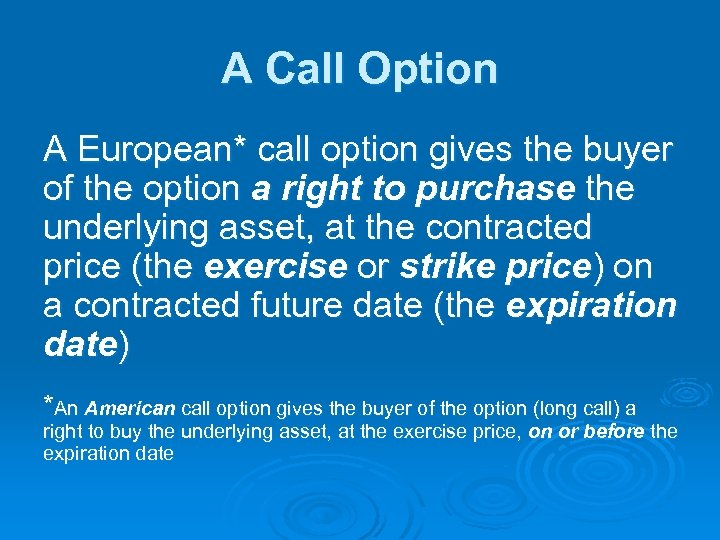A Call Option A European* call option gives the buyer of the option a right to purchase the underlying asset, at the contracted price (the exercise or strike price) on a contracted future date (the expiration date) *An American call option gives the buyer of the option (long call) a right to buy the underlying asset, at the exercise price, on or before the expiration date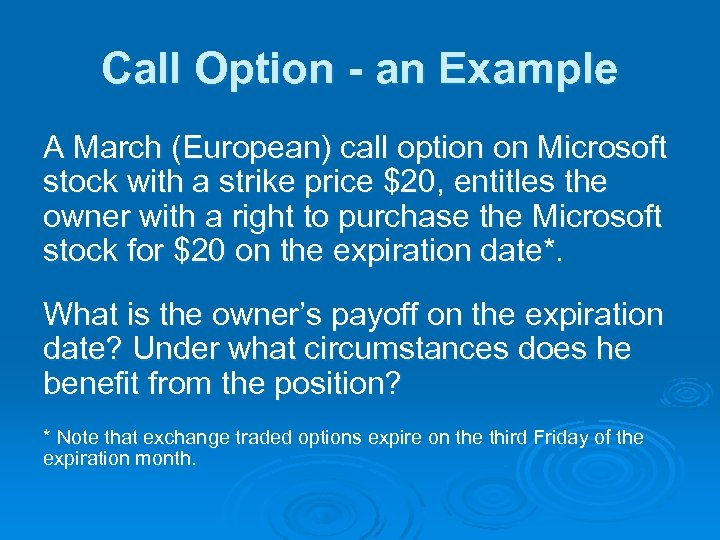Call Option - an Example A March (European) call option on Microsoft stock with a strike price \$20, entitles the owner with a right to purchase the Microsoft stock for \$20 on the expiration date*. What is the owner’s payoff on the expiration date? Under what circumstances does he benefit from the position? * Note that exchange traded options expire on the third Friday of the expiration month.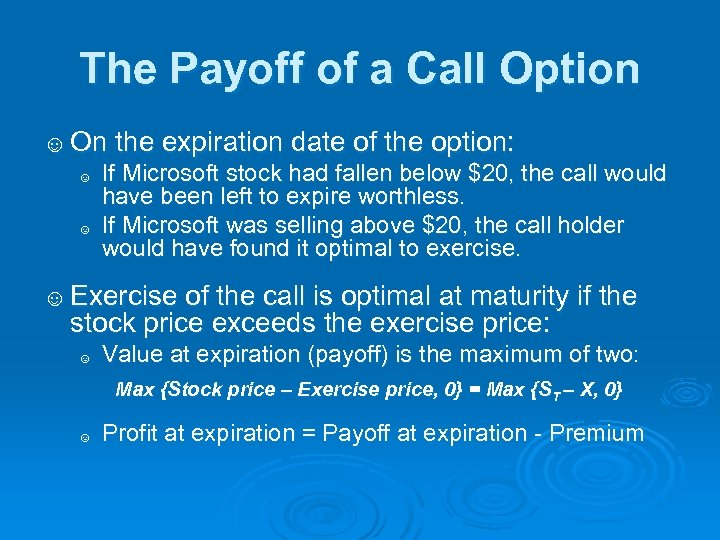The Payoff of a Call Option ☺ On the expiration date of the option: ☺ If Microsoft stock had fallen below \$20, the call would have been left to expire worthless. ☺ If Microsoft was selling above \$20, the call holder would have found it optimal to exercise. ☺ Exercise of the call is optimal at maturity if the stock price exceeds the exercise price: ☺ Value at expiration (payoff) is the maximum of two: Max {Stock price – Exercise price, 0} = Max {ST – X, 0} ☺ Profit at expiration = Payoff at expiration - Premium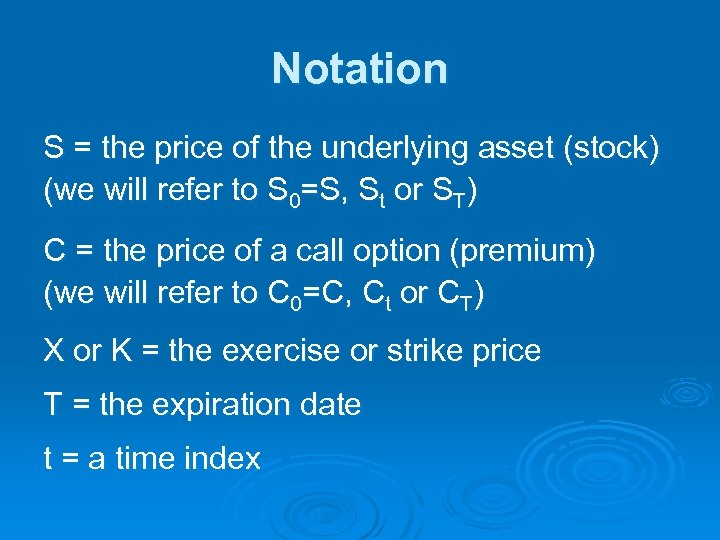Notation S = the price of the underlying asset (stock) (we will refer to S 0=S, St or ST) C = the price of a call option (premium) (we will refer to C 0=C, Ct or CT) X or K = the exercise or strike price T = the expiration date t = a time index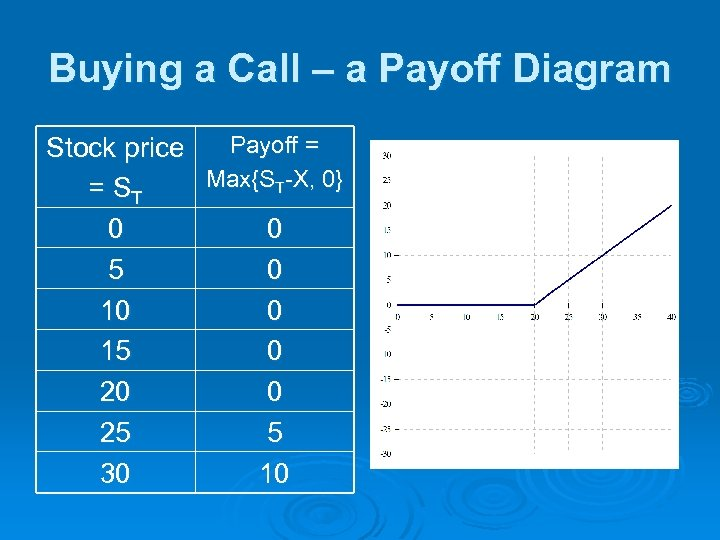Buying a Call – a Payoff Diagram Payoff = Stock price Max{ST-X, 0} = ST 0 0 5 0 10 0 15 0 20 0 25 5 30 10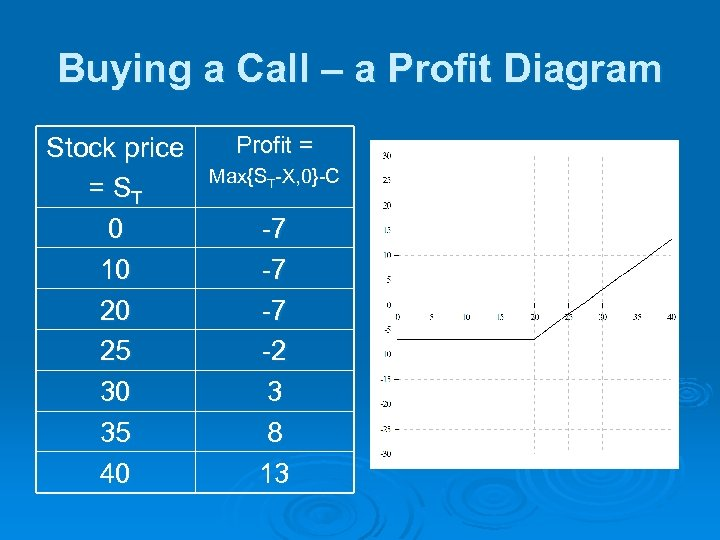Buying a Call – a Profit Diagram Stock price = ST 0 10 20 25 30 35 40 Profit = Max{ST-X, 0}-C -7 -7 -7 -2 3 8 13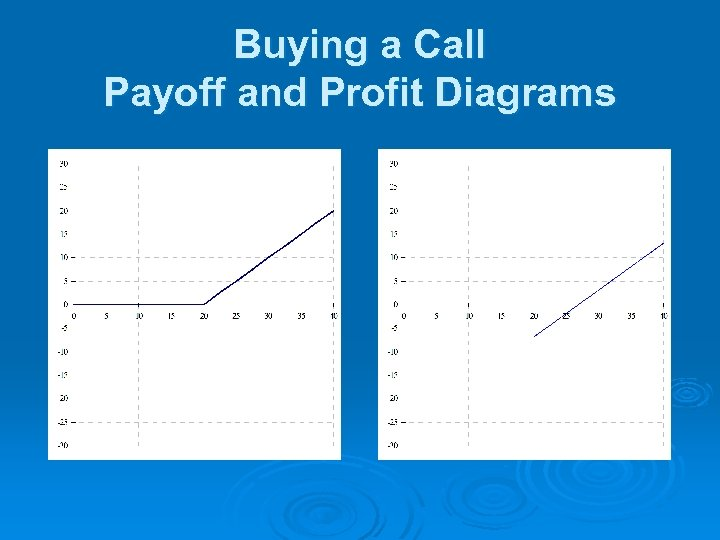Buying a Call Payoff and Profit Diagrams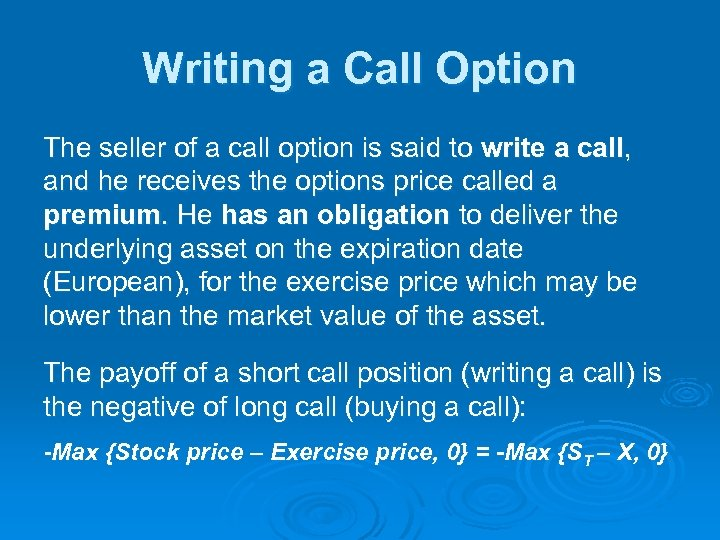Writing a Call Option The seller of a call option is said to write a call, and he receives the options price called a premium. He has an obligation to deliver the underlying asset on the expiration date (European), for the exercise price which may be lower than the market value of the asset. The payoff of a short call position (writing a call) is the negative of long call (buying a call): -Max {Stock price – Exercise price, 0} = -Max {ST – X, 0}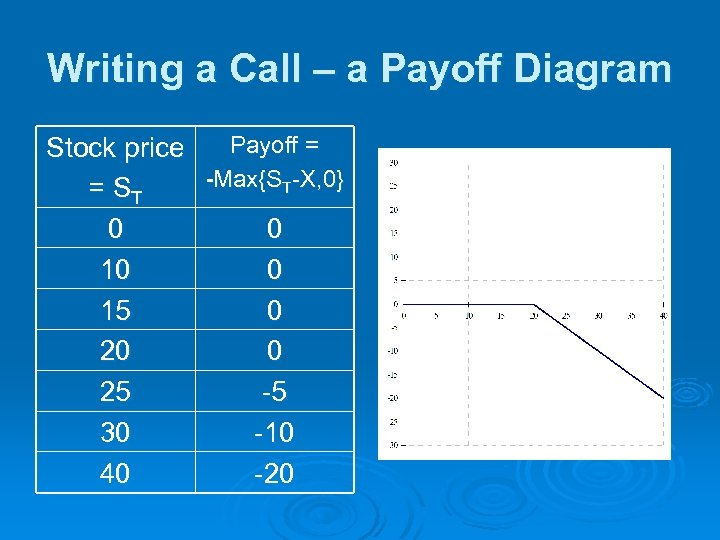Writing a Call – a Payoff Diagram Payoff = Stock price -Max{ST-X, 0} = ST 0 0 15 0 20 0 25 -5 30 -10 40 -20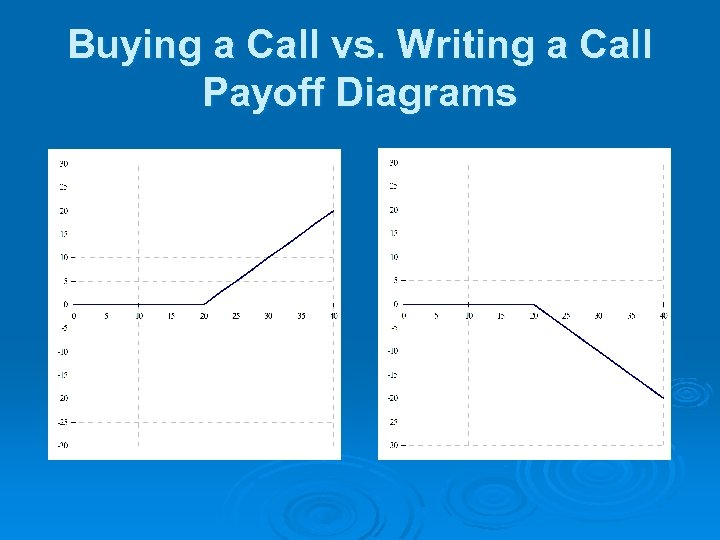Buying a Call vs. Writing a Call Payoff Diagrams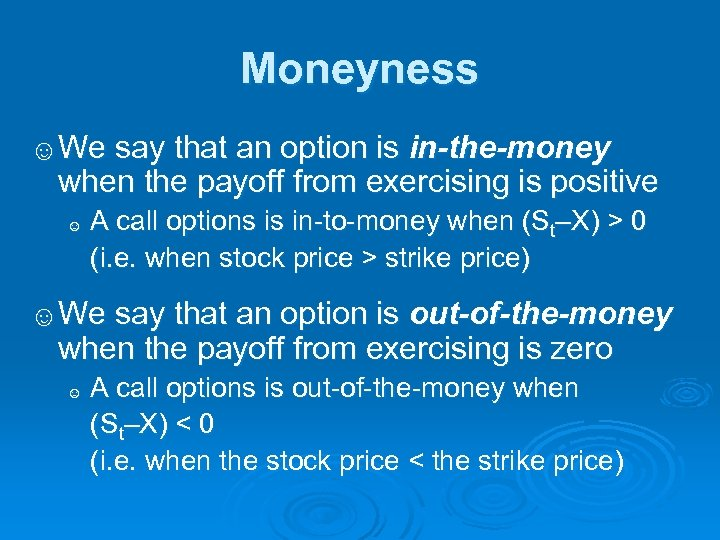Moneyness ☺We say that an option is in-the-money when the payoff from exercising is positive ☺ A call options is in-to-money when (St–X) > 0 (i. e. when stock price > strike price) ☺We say that an option is out-of-the-money when the payoff from exercising is zero ☺ A call options is out-of-the-money when (St–X) < 0 (i. e. when the stock price < the strike price)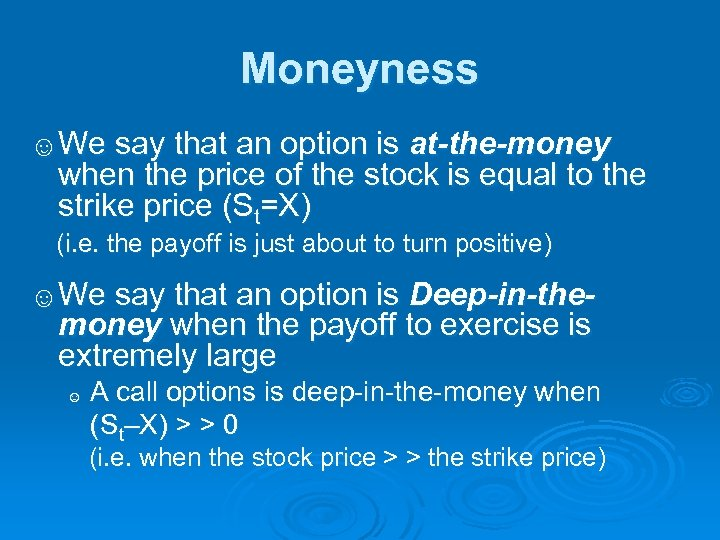Moneyness ☺We say that an option is at-the-money when the price of the stock is equal to the strike price (St=X) (i. e. the payoff is just about to turn positive) ☺We say that an option is Deep-in-themoney when the payoff to exercise is extremely large ☺ A call options is deep-in-the-money when (St–X) > > 0 (i. e. when the stock price > > the strike price)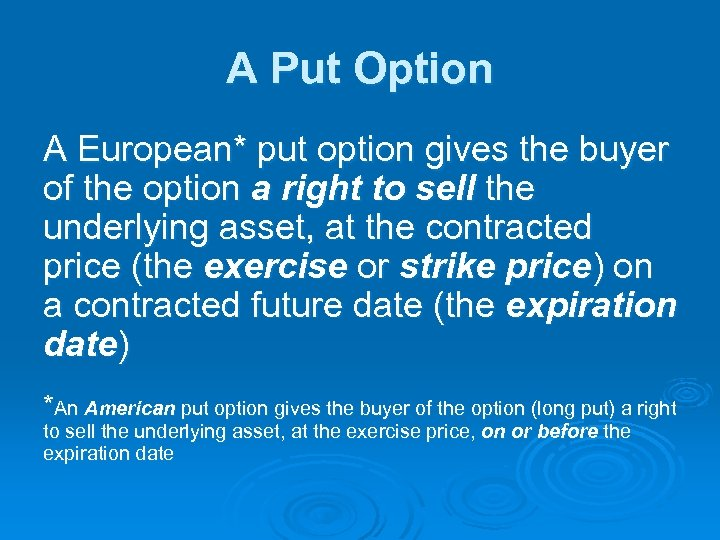A Put Option A European* put option gives the buyer of the option a right to sell the underlying asset, at the contracted price (the exercise or strike price) on a contracted future date (the expiration date) *An American put option gives the buyer of the option (long put) a right to sell the underlying asset, at the exercise price, on or before the expiration date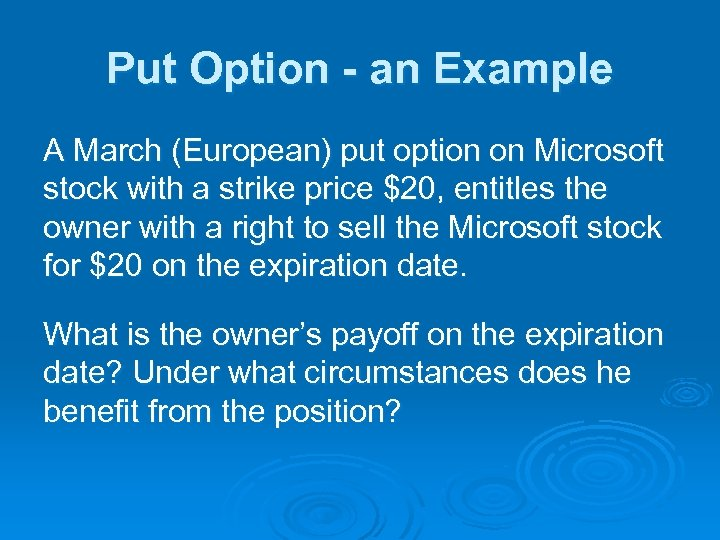Put Option - an Example A March (European) put option on Microsoft stock with a strike price \$20, entitles the owner with a right to sell the Microsoft stock for \$20 on the expiration date. What is the owner’s payoff on the expiration date? Under what circumstances does he benefit from the position?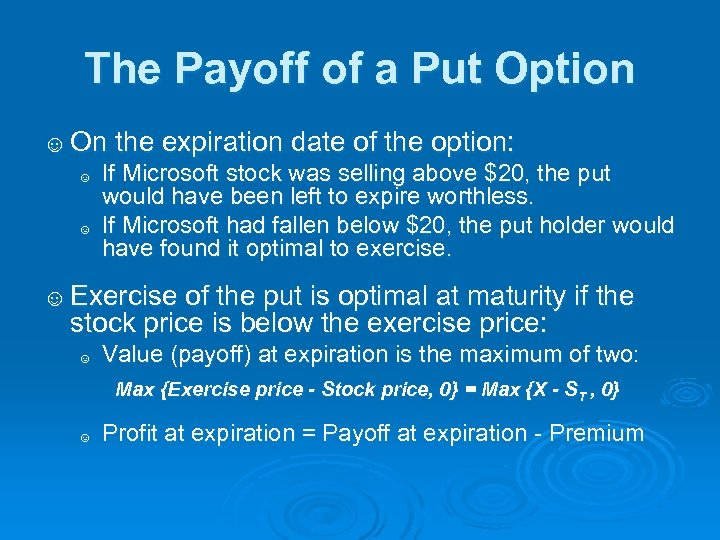The Payoff of a Put Option ☺ On the expiration date of the option: ☺ If Microsoft stock was selling above \$20, the put would have been left to expire worthless. ☺ If Microsoft had fallen below \$20, the put holder would have found it optimal to exercise. ☺ Exercise of the put is optimal at maturity if the stock price is below the exercise price: ☺ Value (payoff) at expiration is the maximum of two: Max {Exercise price - Stock price, 0} = Max {X - ST , 0} ☺ Profit at expiration = Payoff at expiration - Premium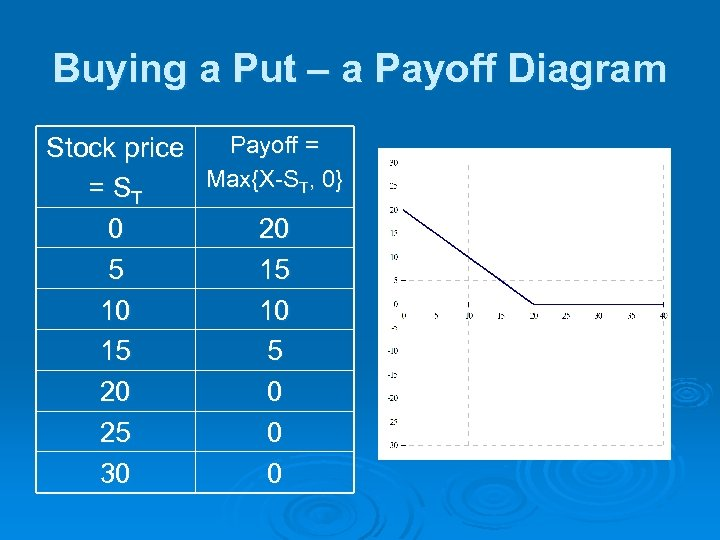Buying a Put – a Payoff Diagram Payoff = Stock price Max{X-ST, 0} = ST 0 20 5 15 10 10 15 5 20 0 25 0 30 0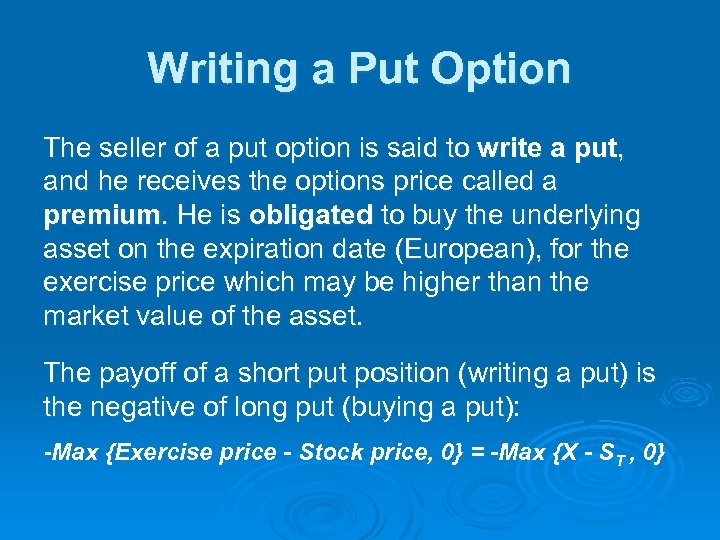Writing a Put Option The seller of a put option is said to write a put, and he receives the options price called a premium. He is obligated to buy the underlying asset on the expiration date (European), for the exercise price which may be higher than the market value of the asset. The payoff of a short put position (writing a put) is the negative of long put (buying a put): -Max {Exercise price - Stock price, 0} = -Max {X - ST , 0}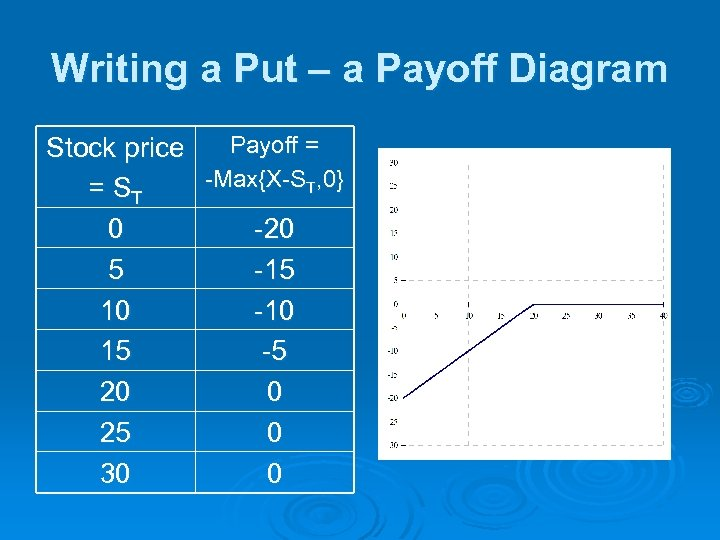Writing a Put – a Payoff Diagram Payoff = Stock price -Max{X-ST, 0} = ST 0 -20 5 -15 10 -10 15 -5 20 0 25 0 30 0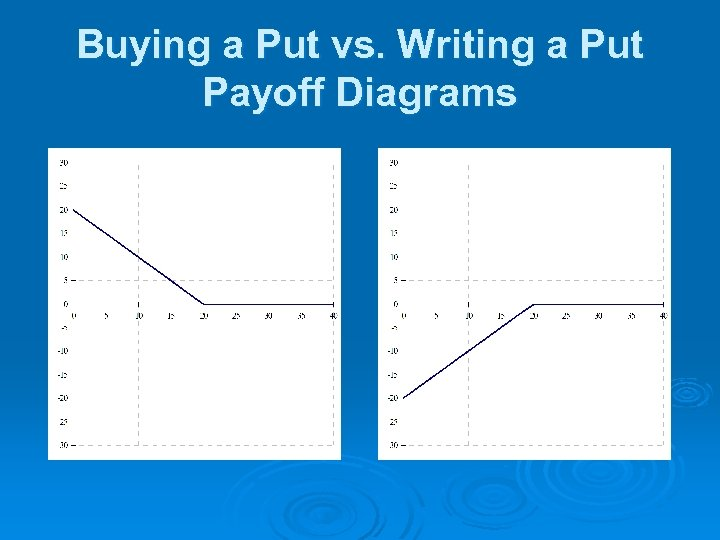Buying a Put vs. Writing a Put Payoff Diagrams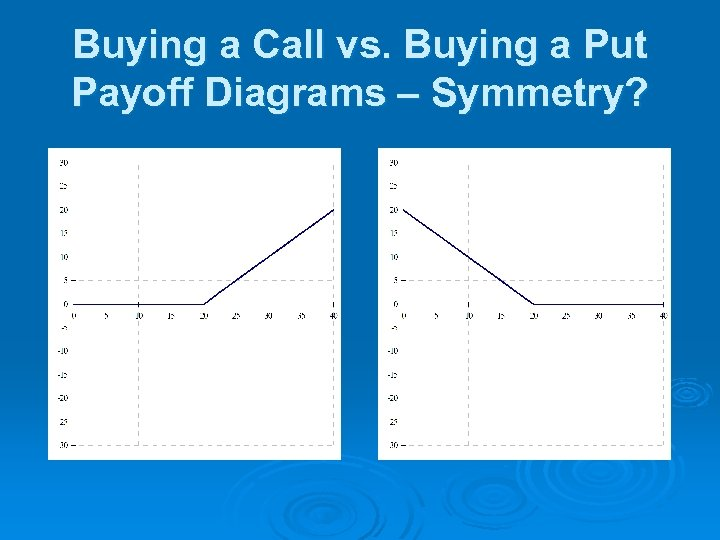Buying a Call vs. Buying a Put Payoff Diagrams – Symmetry?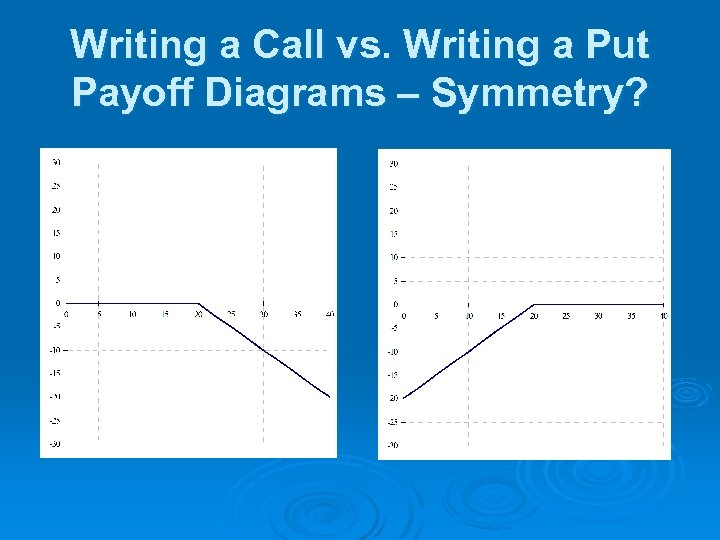Writing a Call vs. Writing a Put Payoff Diagrams – Symmetry?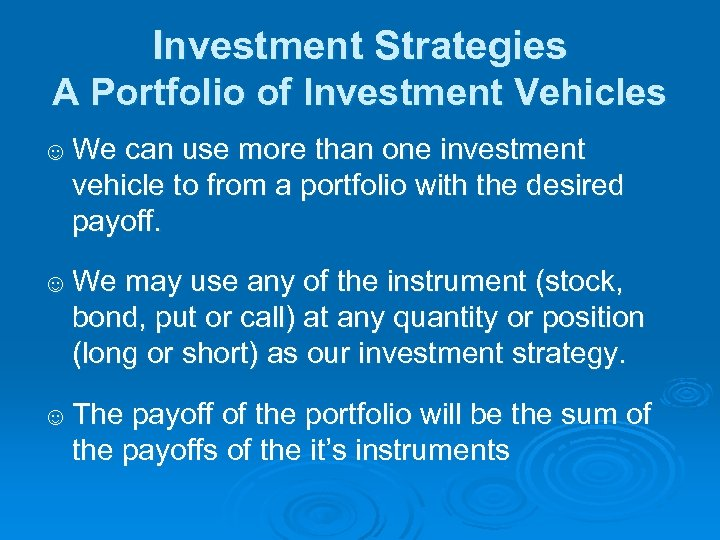Investment Strategies A Portfolio of Investment Vehicles ☺ We can use more than one investment vehicle to from a portfolio with the desired payoff. ☺ We may use any of the instrument (stock, bond, put or call) at any quantity or position (long or short) as our investment strategy. ☺ The payoff of the portfolio will be the sum of the payoffs of the it’s instruments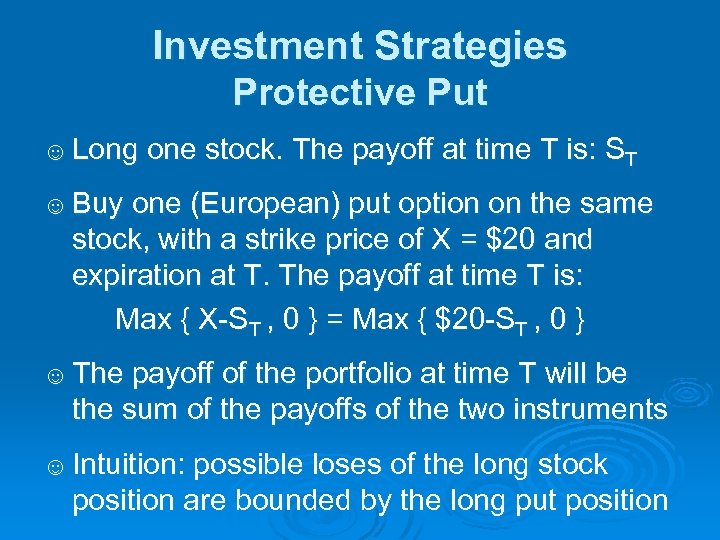Investment Strategies Protective Put ☺ Long one stock. The payoff at time T is: ST ☺ Buy one (European) put option on the same stock, with a strike price of X = \$20 and expiration at T. The payoff at time T is: Max { X-ST , 0 } = Max { \$20 -ST , 0 } ☺ The payoff of the portfolio at time T will be the sum of the payoffs of the two instruments ☺ Intuition: possible loses of the long stock position are bounded by the long put position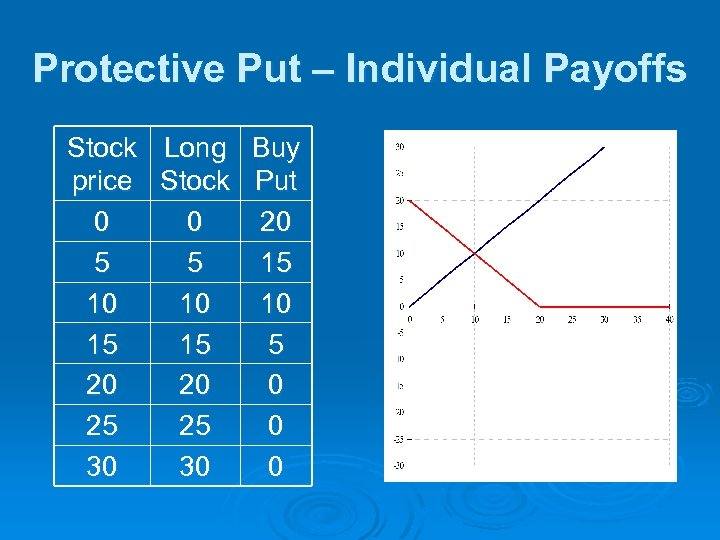Protective Put – Individual Payoffs Stock Long Buy price Stock Put 0 0 20 5 5 15 10 10 10 15 15 5 20 20 0 25 25 0 30 30 0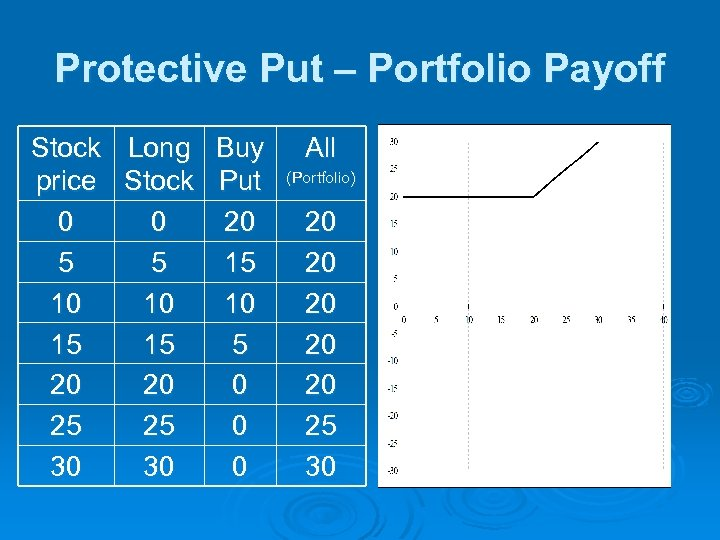Protective Put – Portfolio Payoff Stock Long Buy price Stock Put 0 0 20 5 5 15 10 10 10 15 15 5 20 20 0 25 25 0 30 30 0 All (Portfolio) 20 20 20 25 30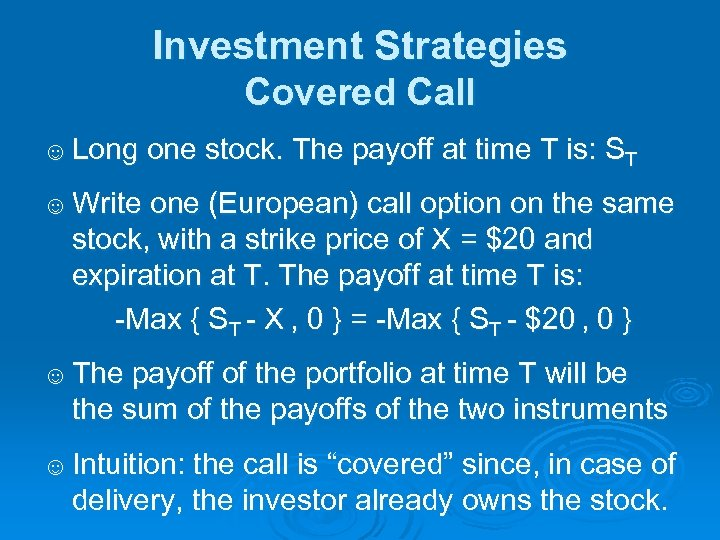Investment Strategies Covered Call ☺ Long one stock. The payoff at time T is: ST ☺ Write one (European) call option on the same stock, with a strike price of X = \$20 and expiration at T. The payoff at time T is: -Max { ST - X , 0 } = -Max { ST - \$20 , 0 } ☺ The payoff of the portfolio at time T will be the sum of the payoffs of the two instruments ☺ Intuition: the call is “covered” since, in case of delivery, the investor already owns the stock.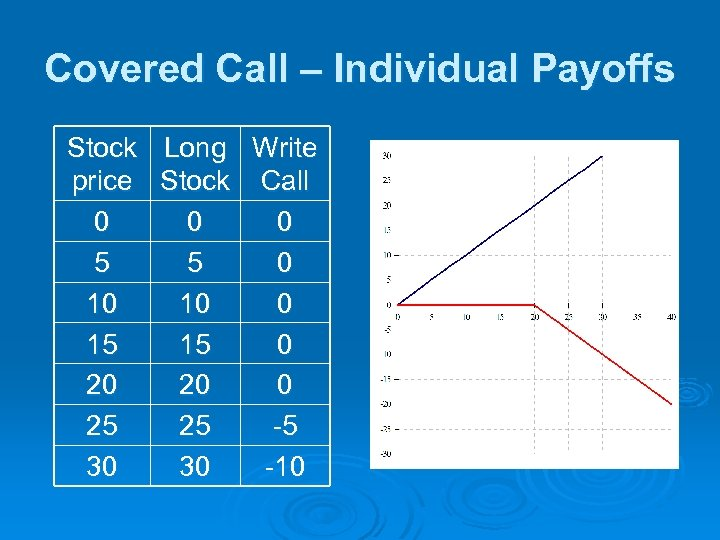Covered Call – Individual Payoffs Stock Long Write price Stock Call 0 0 0 5 5 0 10 10 0 15 15 0 20 20 0 25 25 -5 30 30 -10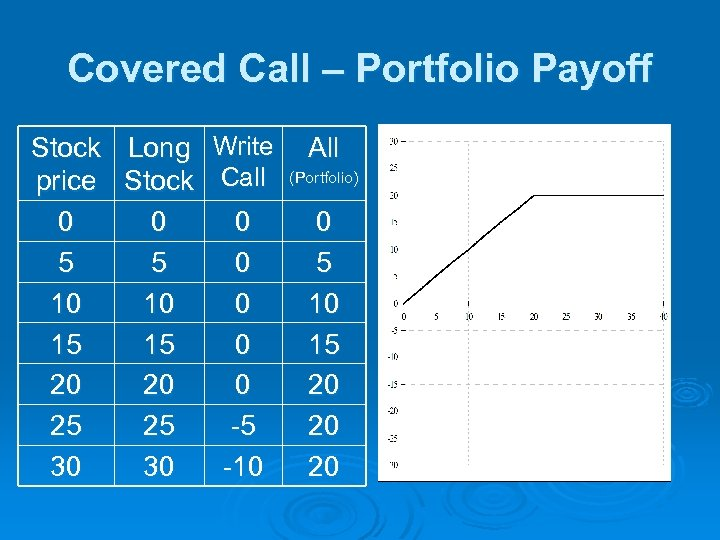Covered Call – Portfolio Payoff Stock Long Write price Stock Call 0 0 0 5 5 0 10 10 0 15 15 0 20 20 0 25 25 -5 30 30 -10 All (Portfolio) 0 5 10 15 20 20 20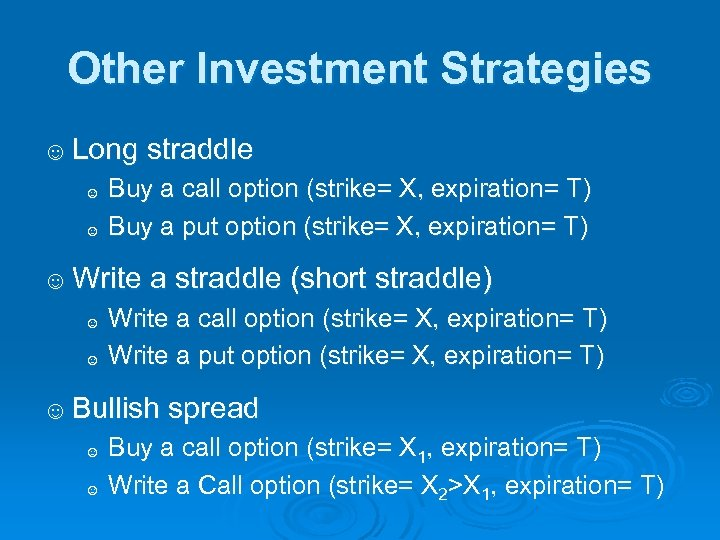Other Investment Strategies ☺ Long straddle ☺ ☺ Buy a call option (strike= X, expiration= T) Buy a put option (strike= X, expiration= T) ☺ Write a straddle (short straddle) ☺ ☺ Write a call option (strike= X, expiration= T) Write a put option (strike= X, expiration= T) ☺ Bullish spread ☺ ☺ Buy a call option (strike= X 1, expiration= T) Write a Call option (strike= X 2>X 1, expiration= T)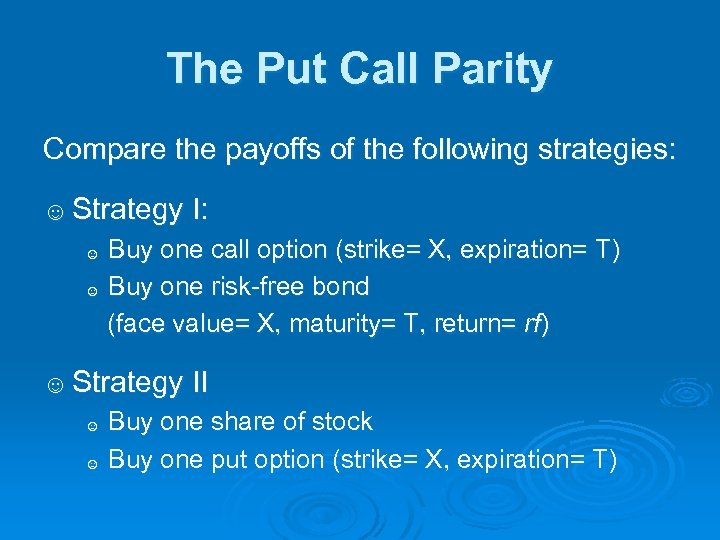The Put Call Parity Compare the payoffs of the following strategies: ☺ Strategy I: Buy one call option (strike= X, expiration= T) ☺ Buy one risk-free bond (face value= X, maturity= T, return= rf) ☺ ☺ Strategy II ☺ ☺ Buy one share of stock Buy one put option (strike= X, expiration= T)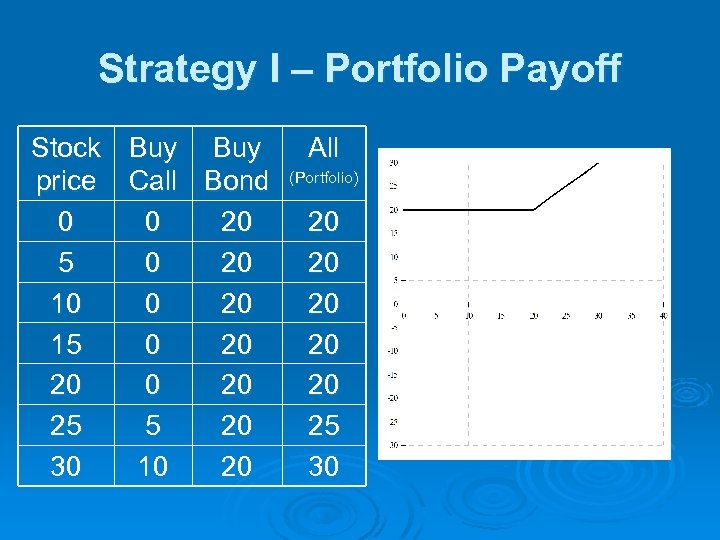Strategy I – Portfolio Payoff Stock Buy price Call Bond 0 0 20 5 0 20 10 0 20 15 0 20 20 25 5 20 30 10 20 All (Portfolio) 20 20 20 25 30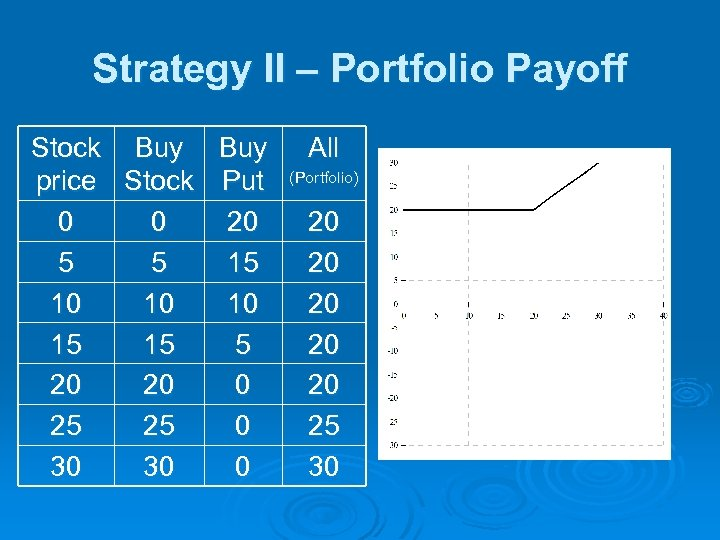Strategy II – Portfolio Payoff Stock Buy price Stock Put 0 0 20 5 5 15 10 10 10 15 15 5 20 20 0 25 25 0 30 30 0 All (Portfolio) 20 20 20 25 30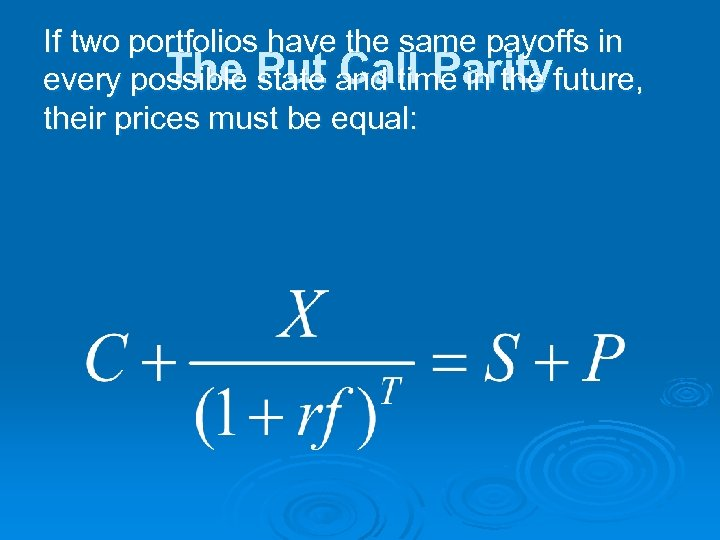If two portfolios have the same payoffs in The state Call Parity every possible Put and time in the future, their prices must be equal: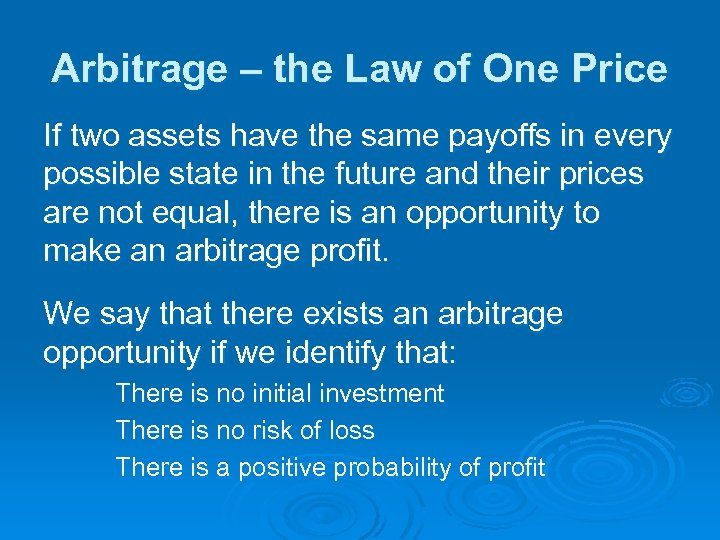Arbitrage – the Law of One Price If two assets have the same payoffs in every possible state in the future and their prices are not equal, there is an opportunity to make an arbitrage profit. We say that there exists an arbitrage opportunity if we identify that: There is no initial investment There is no risk of loss There is a positive probability of profit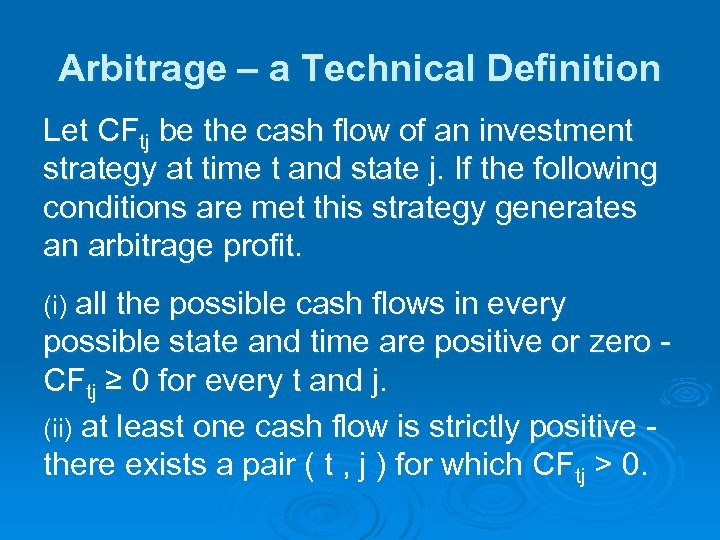Arbitrage – a Technical Definition Let CFtj be the cash flow of an investment strategy at time t and state j. If the following conditions are met this strategy generates an arbitrage profit. all the possible cash flows in every possible state and time are positive or zero CFtj ≥ 0 for every t and j. (ii) at least one cash flow is strictly positive there exists a pair ( t , j ) for which CFtj > 0. (i)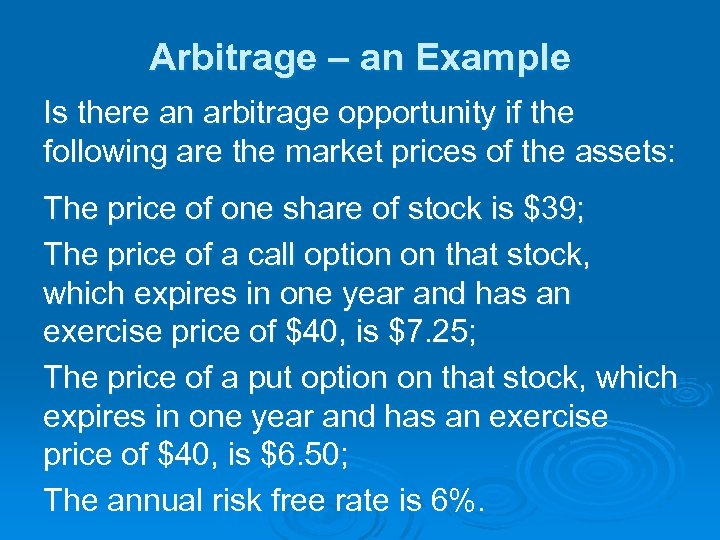Arbitrage – an Example Is there an arbitrage opportunity if the following are the market prices of the assets: The price of one share of stock is \$39; The price of a call option on that stock, which expires in one year and has an exercise price of \$40, is \$7. 25; The price of a put option on that stock, which expires in one year and has an exercise price of \$40, is \$6. 50; The annual risk free rate is 6%.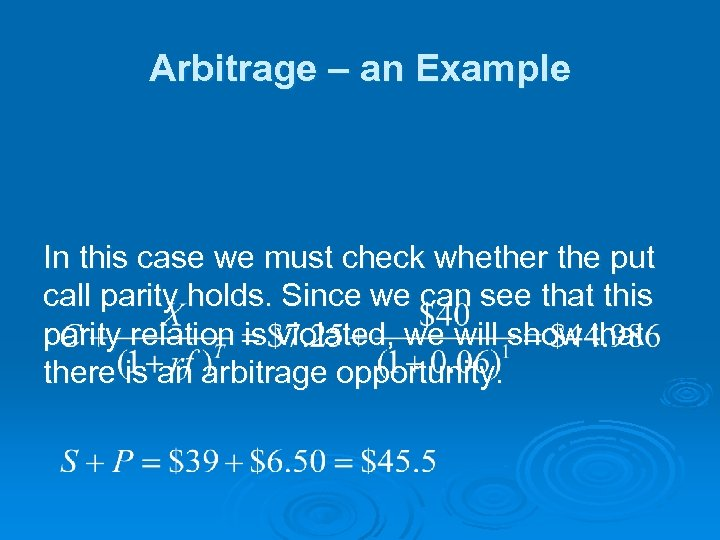Arbitrage – an Example In this case we must check whether the put call parity holds. Since we can see that this parity relation is violated, we will show that there is an arbitrage opportunity.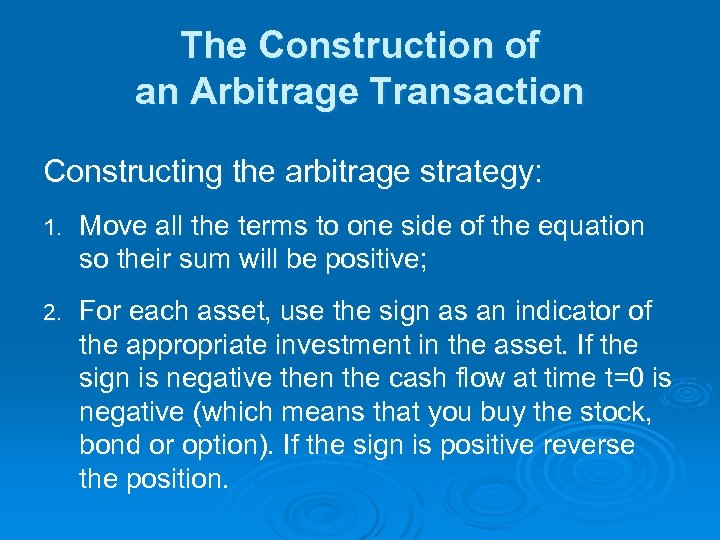The Construction of an Arbitrage Transaction Constructing the arbitrage strategy: 1. Move all the terms to one side of the equation so their sum will be positive; 2. For each asset, use the sign as an indicator of the appropriate investment in the asset. If the sign is negative then the cash flow at time t=0 is negative (which means that you buy the stock, bond or option). If the sign is positive reverse the position.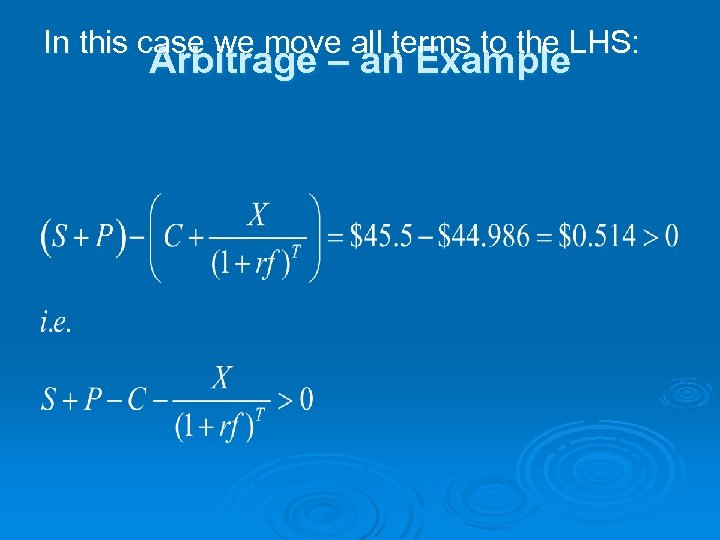In this case we move all terms to the LHS: Arbitrage – an Example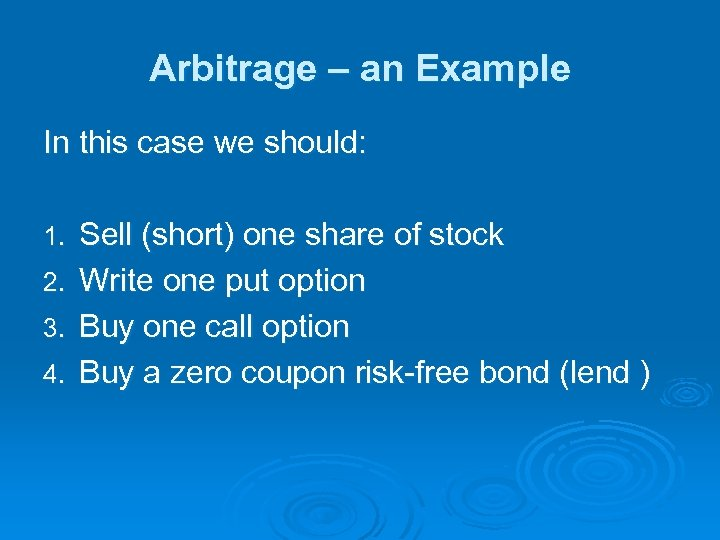Arbitrage – an Example In this case we should: 1. 2. 3. 4. Sell (short) one share of stock Write one put option Buy one call option Buy a zero coupon risk-free bond (lend )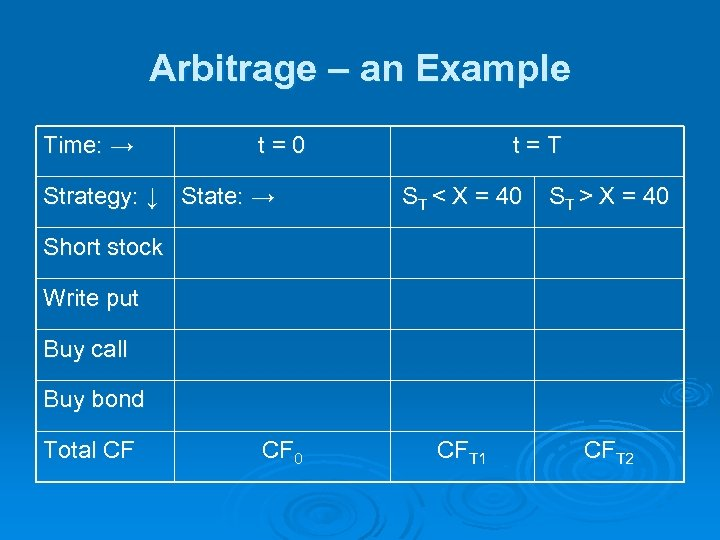Arbitrage – an Example Time: → t=0 Strategy: ↓ State: → t=T ST < X = 40 ST > X = 40 CFT 1 CFT 2 Short stock Write put Buy call Buy bond Total CF CF 0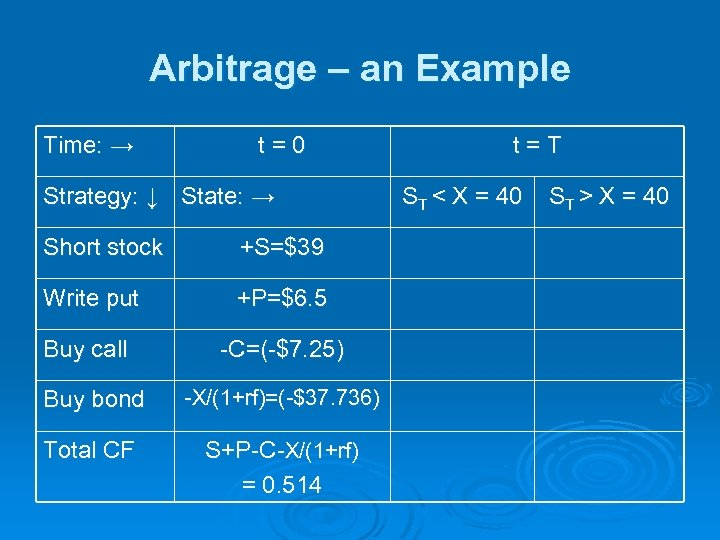Arbitrage – an Example Time: → t=0 Strategy: ↓ State: → Short stock +S=\$39 Write put +P=\$6. 5 Buy call -C=(-\$7. 25) Buy bond -X/(1+rf)=(-\$37. 736) Total CF S+P-C-X/(1+rf) = 0. 514 t=T ST < X = 40 ST > X = 40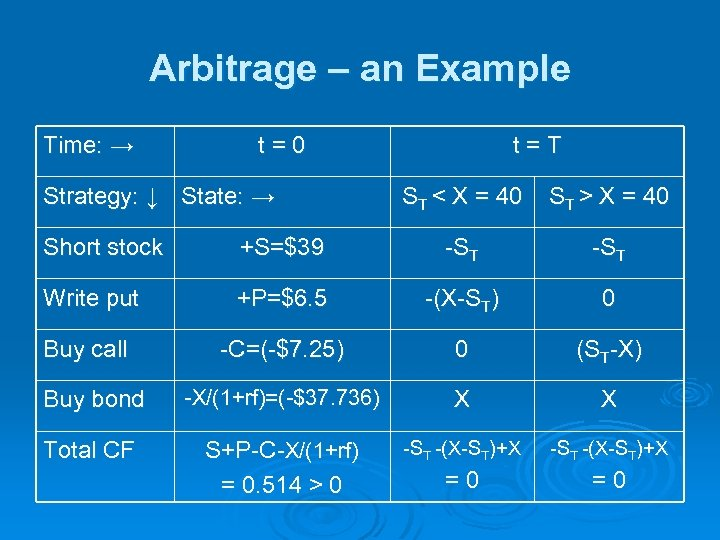Arbitrage – an Example Time: → t=0 Strategy: ↓ State: → t=T ST < X = 40 ST > X = 40 Short stock +S=\$39 -ST Write put +P=\$6. 5 -(X-ST) 0 Buy call -C=(-\$7. 25) 0 (ST-X) Buy bond -X/(1+rf)=(-\$37. 736) X X Total CF S+P-C-X/(1+rf) = 0. 514 > 0 -ST -(X-ST)+X =0 =0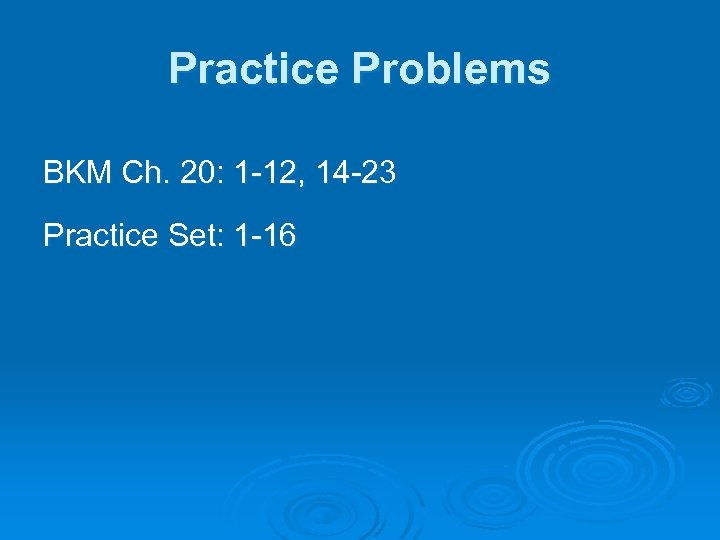Practice Problems BKM Ch. 20: 1 -12, 14 -23 Practice Set: 1 -16